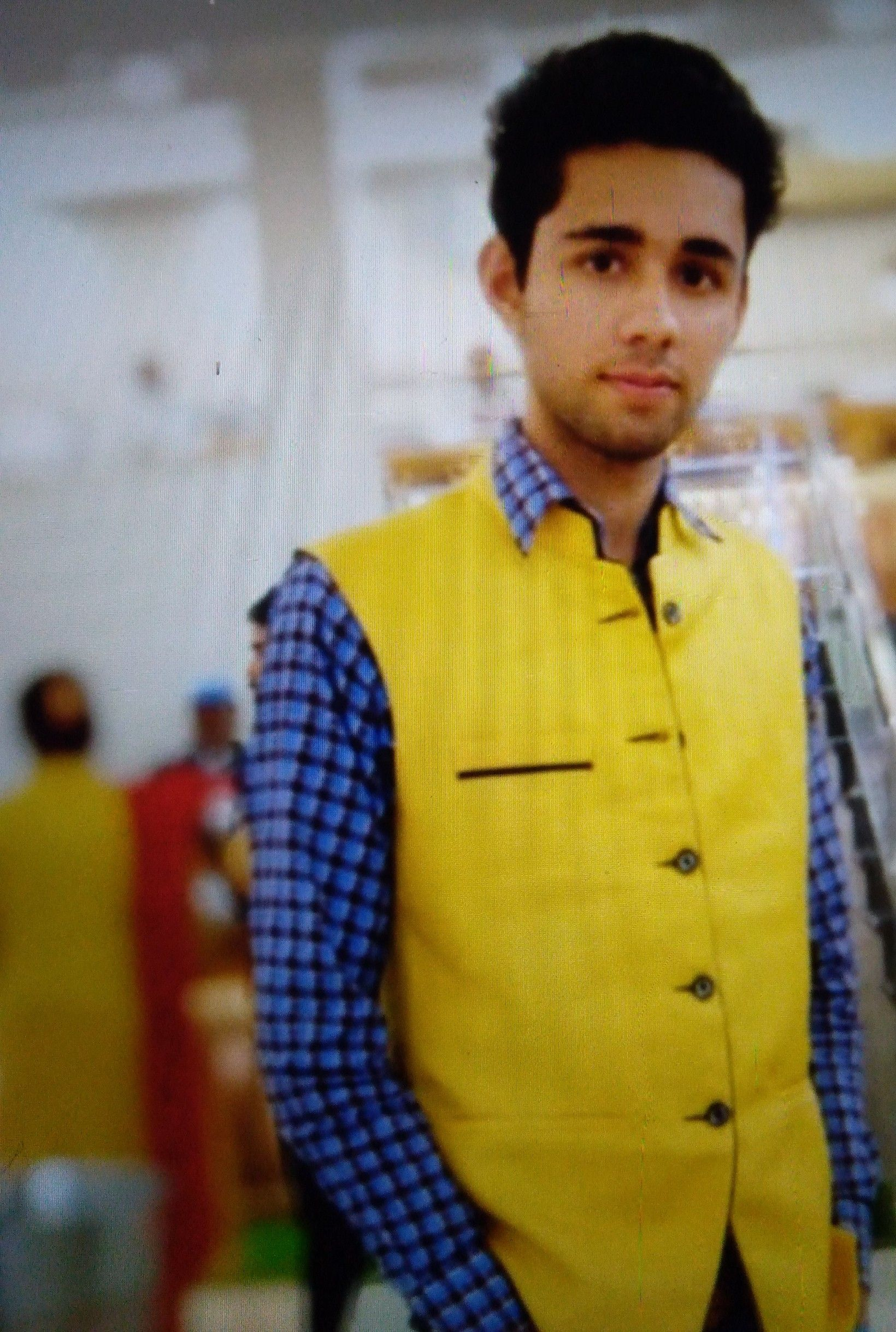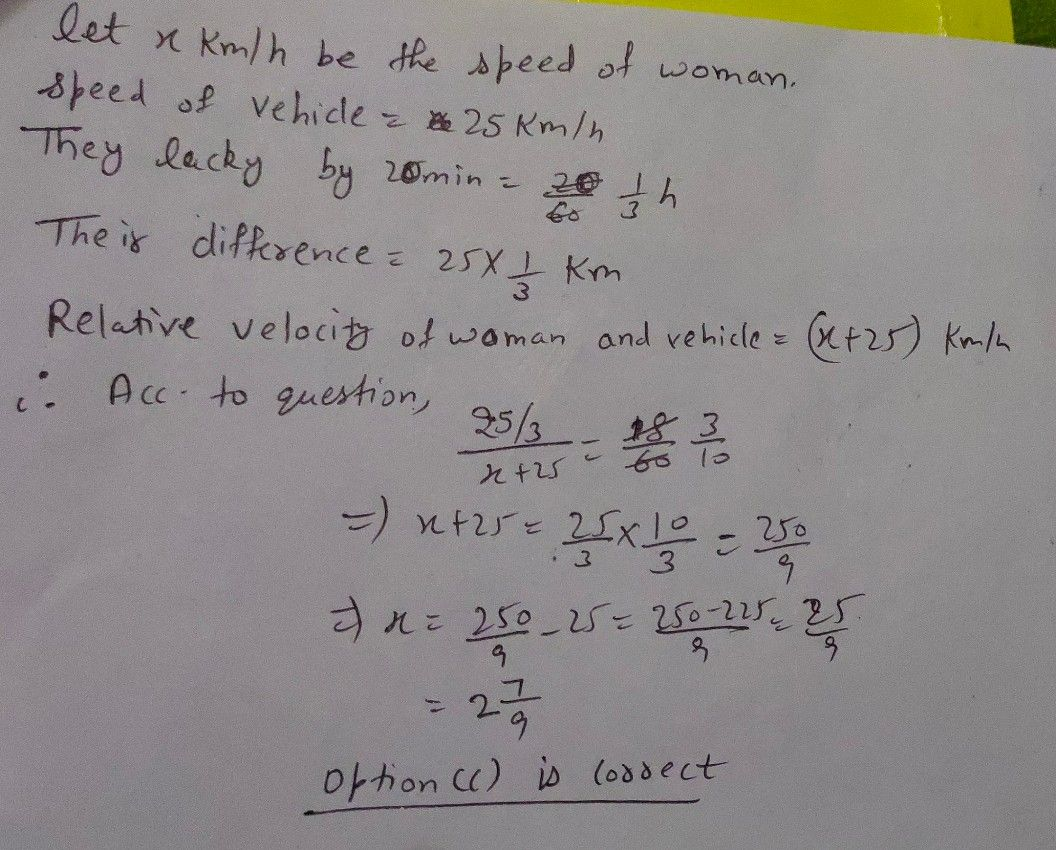Symbol
ProblemCan you answer this? $20$ $25$ $18$ $\left($ $\left(A\right)$ $A\right)2$ $21frac\left(5\right)\left(9\right)$ \) $\left(B\right)$ $B\right)$ $1\left(211$ $\left(C\right)$ $1\left(21$ $21+rac\left(7\right)+9\right)$ \) $\left(D\right)$ $1\left(2\right)$ 2\frac{8}{9} $ac\left(8\right)\left(9\right)$ \) $9:18PM\sqrt{}$
1st-6th grade
Algebra
Search count: 1,480
SolutionQanda teacher - NISHANTStudent
sir g hindi me solve nhi ho sakta h. or thoda samaj me nhi aa reha hQanda teacher - NISHANT
x mahila ki speed h
dono vahno ki speed 25kh/h h
km/h*
vahno k beech ki duri =25/3 km
mahila aur vahn ki relative speed 25+X km/h hogi
hame pata h ki mahila vahn ki 18 min me milti h
isilie duri /speed = samay
rakh dia
or answer aa gyaStudent
ok sir, thanks g